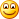## Saturday, July 9, 2011

### Tech Qu: Find the numbers that occurs an odd number of times in a huge array of integers.

This is a more complex version of a reported Amazon question, where there is only a single one. There are two questions based on constraints:

• Minimize the time to get the array of numbers.
• Minimize the memory requirements.

## Minimize the time to get the array of numbers: Step 1

The first step of the solution is to use two bit maps :

1. Map A is Int.MaxValue +1 in size
2. Map B is Int.MinValue in size

Both are initialized to zero (the default). Walk the array.

• If not negative, toggle Map A
• If negative, take the negative value (you should raise the issue that the language should be tested for the fastest way to do this, i.e. Math.Abs(), using binary operations, x=-x, etc).
• NOTE: We could use 1 bitmap, but this means that we will need to do an arithmetic operation for 50% of the array. In general bit operations perform better, hence the design should be bit-operation centric.

Once the array has been walked, then walk the bitmaps for non-zero values.  This gets a solution but it is not the fastest way. Magnitude is O(n)

## Minimize the time to get the array of numbers: Step 2

Step two is to split the array and hand each array to a different thread (good time to show off your multiple threading expertise). Each thread hands back the two bitmaps. The bitmaps are then XOR and then walk the bitmaps for non-zero values.

This is a better solution, but is cannot be faster than Step 1 Time/Number of Cores in the machine.

Magnitude is O(n/cores) i.e. still  O(n)

## Minimize the time to get the array of numbers: Step 3

Instead of handing off to different threads, toss portions of the array into a cloud of CPUS (infinitely large potentially!).  Once a portion have been completed it is returned. The bitmaps are then XOR and then walk the bitmaps for non-zero values.  The size of the portions may need to be determine experimentally (i.e. how long does it take to move the array to the cloud and return the bitmap is a significant factor). If the optimal sizing is N items in an array shipped to the cloud, then the time saving for an array of M is approximately N/M less. This actually results in a solution that is O(1).  You can arbitrarily increase the size of the array with the processing time being relatively constant. The time to start the cloud components is O(n), but that is expected to be very minor compared to the time to walk the array which is O(1).

To speed operation completion, you may toss the same array segment to two or more cloud CPUs, thus reducing your variation of time and shifting expected time towards the fastest time of any cloud CPU.

## Minimize the memory requirements

This solution is not fast, but it is compact.

• Sort the array (best time is of the order O( log2 n * n ) see wikipedia).
• Walk the resulting order array to determine the numbers that occur an odd number of times.

## Bottom Line

The difference between finding a solution that is close to O(1) instead of O( log2 n * n ) or  O(n) is significant. The above solution is cloud-centric, which for an Amazon (or Azure) question is likely a better solution because it shows that the person can think in the cloud!